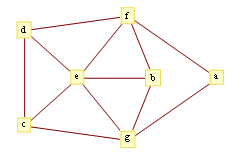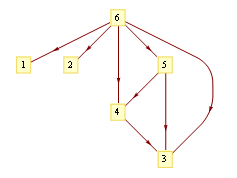GraphPlot. Mathematica 6.0 (2006)

A multilevel algorithm using octree/quadtree data structure to efficiently layout even very large graphs with high quality, based on this paper. The resulting function is Mathematica's GraphPlot, as well as GraphPlot3D, LayeredGraphPlot, TreePlot functions. Examples:

In:= GraphPlot[{"d" -> "c", "e" -> "b", "e" -> "c", "e" -> "d", "f" -> "a",
"f" -> "b", "f" -> "d", "f" -> "e", "g" -> "a", "g" -> "b",
"g" -> "c", "g" -> "e"}, VertexLabeling -> True]

Out:=In:= LayeredGraphPlot[{4 -> 3, 5 -> 3, 5 -> 4, 6 -> 1, 6 -> 2, 6 -> 4,
6 -> 5, 6 -> 3}, VertexLabeling -> True]